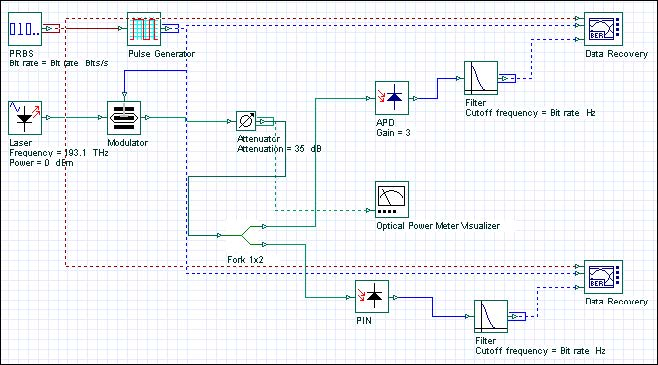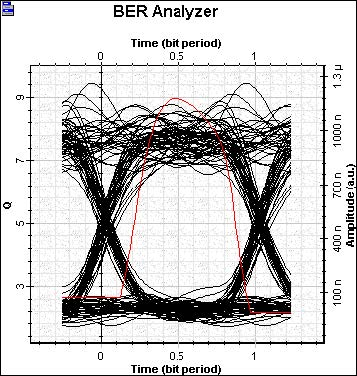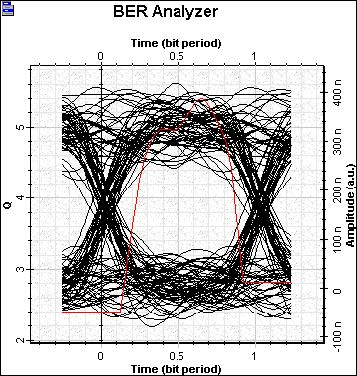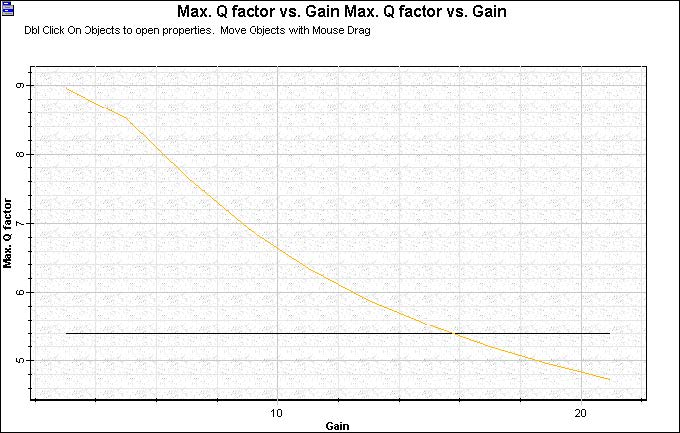Optical receivers with APD generally provide a higher SNR for the same incident optical power. The improvement in the SNR is due to the internal gain that increases the photocurrent by the multiplication factor M.Figure 1: Receiver PIN x APD

The APD photodetector systems (see Figure 2) has a Q factor higher that the one with PIN photodetector for a multiplication factor of 3 (see Figure 3).Figure 2: APD Q FactorFigure 3: PIN Q Factor

If we increase the multiplication factor, there is a point at which the shot noise degrades the system performance, therefore, it is important to find the optimum APD gain.

If you run the same simulation and vary the value of the multiplication factor, you can see the evolution of the Q factor in Figure 4.Figure 4: Q factor x APD Gain

For a gain higher of 16, there is no advantage to using the APD, because it will not improve the receiver sensitivity.